9. RAY OPTICS AND OPTICAL INSTRUMENTS

Q.1. (a) When a low flying aircraft passes overhead, we sometimes notice a slight shaking of the picture of our TV screen. Explain.
(b) Thin films such as soap bubble or a thin layer of oil on water show beautiful colours when illuminated by white light. Explain.

Ans ⇒ (a) Interference in the signal received by the antenna is due to temporary reflection of the signal by the plane passing overhead.

(b) In the thin films, the interference occurs between the wave reflected by the upper and the lower surface. The wave reflected from the lower surface undergoes refraction too, which depends upon the wavelength. Hence, coloure fringes are seen.

Q.2. Draw a labelled ray diagram to show the image formation in a refracting type astronomical telescope. Why should the diameter of the objective of a telescope be large?

Ans ⇒ The diameter of the objective should be large in order to gather maximum R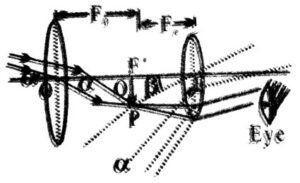Q. 3. Name the device for producing polarised light. How does the intensity of transmitted light depend upon the angle between the polariser and the analyser ? Show it graphically.

Ans ⇒ Calcite Prism or Nicol Prism:
According to Malus law, when a completely plane polarised light is incident on an anlyser, the intensity I of the transmitted lightis proportional to the square of cosine of the angle qbetween the planes of transmissionof analyser and polariser.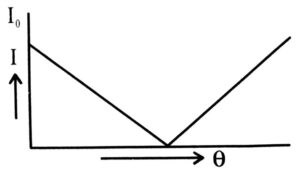So. I = I0 cos2θ lo represent maximum intensity of the transmitted light figure shows the variation of intensity (I) with θ.

Q.4. How does diffraction limit the resolving power of an optical instrument ?

Ans ⇒ Resolving power of telescope is given by R.P. = 1/dθ, where dθ is the angular separation between two objects.
Due to diffraction at a circular aperture, a covering lens cannot form a point image of an object rather it produces a brighter disc surrounded by dark and bright rings. This is called Diffraction pattern. Each point on the object gives rise to separate diffraction pattern. If two points are very lose, then central maxima may get superimposed on each other and then the two images cannot be distinguished. They are said to be unresolved. So, diffraction limits the resolving power of an optical instrument.

Q.5. Derive the relationship between the refractive index and critical angle for a given pair of media.

Ans ⇒ Critical angle is the angle of incidence in the denser medium for which angle of refraction is of 90° in the rarer medium. According to Snell’s law: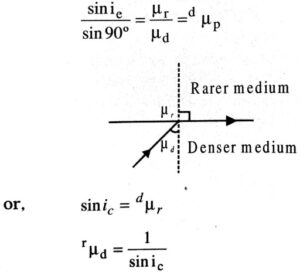Q.6. A ray of light while travelling from denser to rarer medium undergoes total internal reflection. Derive the expression for the critical angle in terms of the speed of light in the respective media.

Ans ⇒ According to Snell’s law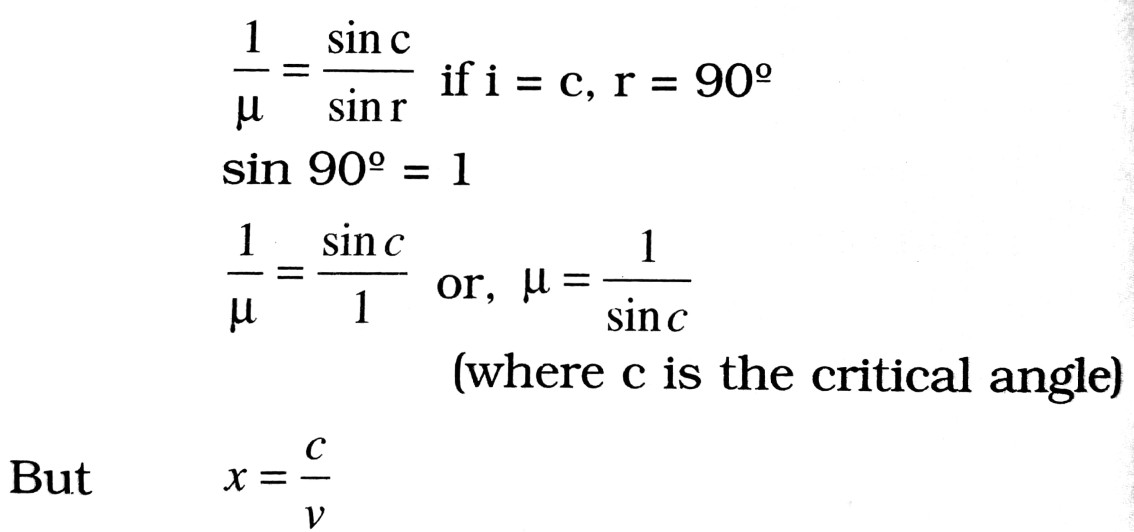where c is the velocity of light = 3 x 108 m/sec. and ‘v’ is the speed of light in the medium.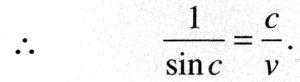Q.7. A ray of light while travelling from a denser to rarer medium undergoes total internal reflection. Derive the expression for the critical angle in terms of the speed of light in the respective medium.

Ans ⇒ According to Snell’s law, the refractive index of glass with respect to air is given by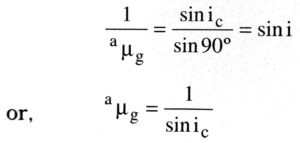Now v is the speed of light in respective medium and c is the speed of light in vacuum = 3 x 108 m/Sec.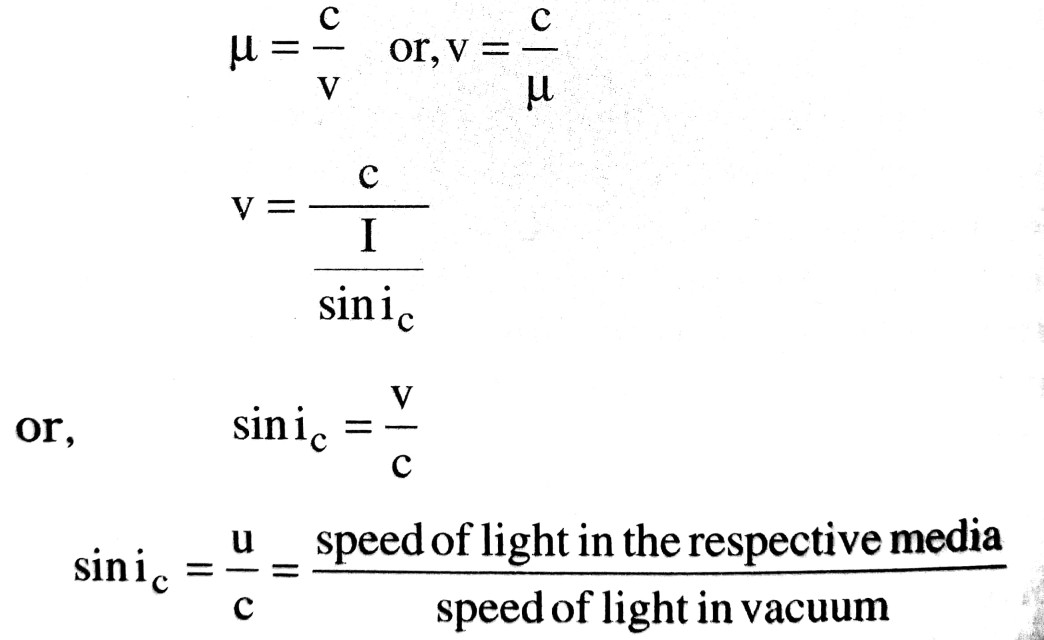Q.8. Explain with reason, how the resolving power of an astronomical telescope will change when (i) frequency of incident light on the objective lens is increased, (ii) focal length of the objective lens is increased, (iii) aperture of the objective lens is halved.

Ans ⇒ (i) Resolving power of telescope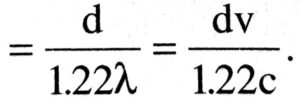Thus, when frequiency of incident light is increased the resolving power increases.

(ii) On increasing the focal length of the objective, the magnifying power of the teliscope increases and hence the revolving power also decreases.

(iii) When aperture of the objective is made hall, the quantity of light reaching the image point is decreased and the resolving power also decreases.

Q.9. Give reason for the following observations made from earth:
(i) Sun is visible before the actual sunrise.
(ii) Sun looks reddish at sunset.

Ans ⇒ (i) The rays of light incident on the layers of atmosphere get refracted. Since, the density atmosphere goes on decreasing, therefore, aiter refraction through the layers of atmosphere the light suffers total internal reflection and reaches the earth. Thus, although the sun is not rise, yet it is visible lo the person on the earth.

Apparent position of sun :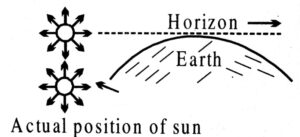(ii) The sun appears red at sun set because in; this position of the sun, light travels a much larger distance through the atmosphere. Hence, blue light is scattered away and light reaching the observer is only red, which is least scattered. This is why sun looks reddish at sunset.

Q.10. List some advantages of a reflection telescope, especially for high resolution astronomy.

Ans ⇒ Advantages of a reflecting telescope :
(i) No chromatic aberration due to the objective because only reflection is involved.
(ii) Spherical aberration reduced by using a mirror of the shape of paraboloid.
(iii) Brighter image than in a refracting telescope of equivalent size because in the latter case, intensity of light is partially lost due to reflection and absorption by the objective lens glass.

(iv) High resolution achieved by using a mirror of large aperture which is exist to support than a lens of the same aperture.

Q.11. A converging and a diverging lens of equal focal lengths are placed coaxially in contact. Find the power and focal length of the comb ination.

Ans ⇒ Let f and -f be the focal length of the converging and diverging lenses respectively.                      Power of the combination will be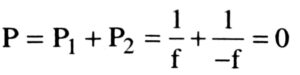Find length of the combination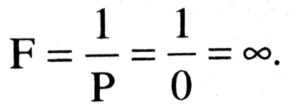Q.12. Draw a labelled ray diagram of a reflecting type telescope. Write its any one advantage over refracting type telescope.

Ans ⇒ Reflecting type telescope was designed by Newton. It is used for observing distant stars.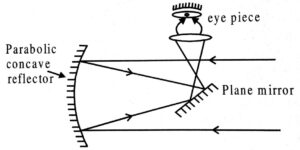Advantage of Reflecting type telesope over Refracting type telescope :
(i) There is no chromatic aberration as the objective is mirror.
(ii) We get the brighter image as compared to the refracting type telescope.
(iii) Spherical aberration is also reduced.

Q.13. A region is illuminated by two sources of light. The intensity I at each point is found to be equal to I1 + I2, where I1 is the intensity of light at the point when sources 2 is absent. I2 is similarly defined. Are the sources coherent or incoherent ? Explain.

Ans ⇒ Intensity at a point due to two sources is given by
I = I1 + 12
Here the two sources have different frequencies and hence the interference term is zero.
Thus the two sources are incoherent because at any frequency, the radiation from one has no stable relation with other.

Q.14. State Brewster law. Using this law prove that at the polarising angle of incidence, the reflected and transmitted says are perpendicular to each other.

Ans ⇒ According to Brewster’s law “the tangent of the angle of polarisation for a transparent medium is equal to the refractive index of the medium.”

That is, θ = tan ip
Proof: From Snell’s law of refraction. We have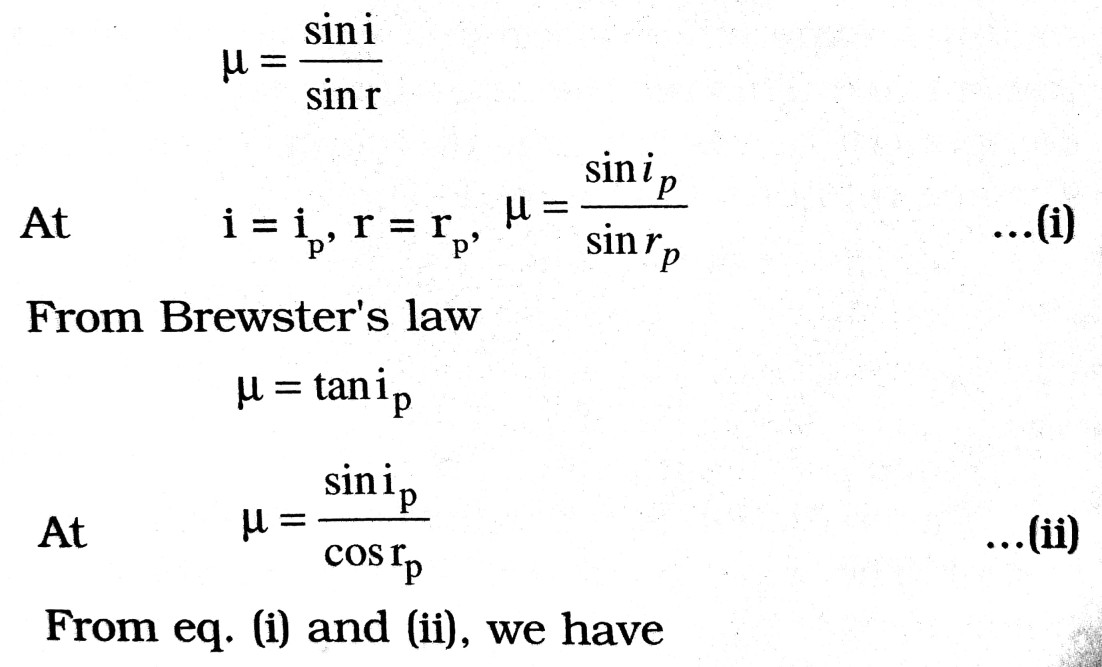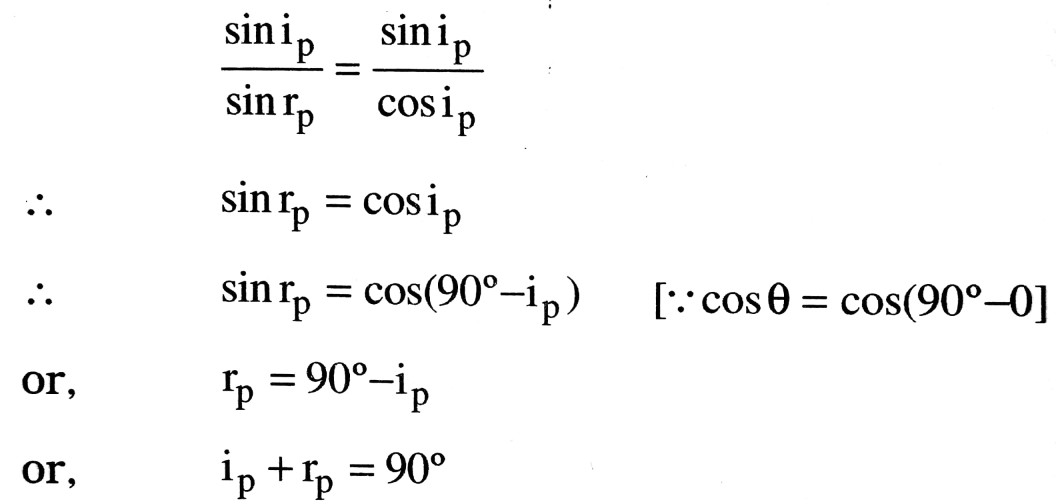That is the reflected and transmitted rays are perpendicular to each other.

Q.15. What are polaroids ? Give their two uses,

Ans ⇒ Polaroids : A polaroid is commercial substance which makes use of dichroism to produce an intense beam of plane polarised light.
When a paste of quinine iodosulphate made in nitrocellulose is squeezed out through a fine slit, the needle-shaped crystal of quinineiodosulphate align themselves parallel to their optic axis, producing them polarising sheet commercially known as polaroid.

Uses of polaroids :
(i) In sun glasses and camera filters : Sun glasses and camera filters are made of polarising sheets to reduce the glare of light produced by reflection from shiny surface such as water surface.

(ii) In wind screens : The wind screens and car head lights of motorcars are fitted with polaroid films with their axes inclined at 45° to the horizontal. When two cars approach each other from opposite directions, the transmission planes of their wind screen will be perpendicular to each other to the glare of their head lights is completely eliminated. Each driver sees the road by light sent by his own car.

Q.16. Explain how Newton’s corpuscular theory predicts the speed of light in a medium, say water, to be greater than the speed of light in vacuum. Is the prediction confirmed by experimental determination of the speed of light in water ? If not, which alternative picture of light is consistent with experiment ?

Ans ⇒ In Newton’s corpuscular picture of refraction particles of light incident from a rarer to a denser medium experience a force of attraction normal to the surface. This results in an increase in the normal component of velocity but the component along the surface remains unchanged, Therefore,
c sin i = μsinr,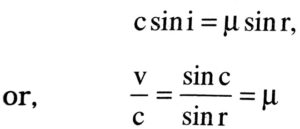Since μ > 1, Therefore v > c
The prediction is opposite to the experiment result (v < c). The wave picture of light is consistent with experiment.

Q.17. Electron microscope is considered better instrument than optical microscope. Give one single reason.

Ans ⇒ In electron microscope instead of visible light we use electron rays whose wavelength is much smaller than that of the visible light. From De-Broglie hypothesis,
λ = h/mv. In electron microscope the angle of cone is also smaller than the angle of cone is simple microscope. A good microscope used in visible light has magnifying power of 2000 nearly where as with electron microscope a magnification of the order of 105 can be obtained.

Q.18. Use mirror formula to show that the virtual image formed by a convex mirror is always diminished in size and is located between the focus and the pole.

Ans ⇒ From the mirror formula,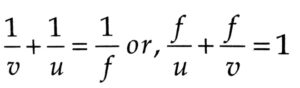Or,        vf + uf – uv = 0 Or, uv – vf = 0
Adding f2 on both sides we get,
vu – uf – vf + f2 = f2
Or,          u(v-f) – (v-f) = f2
∴             (u-f) (v-f) = f2

Let the distance of object and distance of image from the principal focus be x and y respectively.
Then, x = u – f and y = V – f and then from the above equation. xy = f2
This above equation is called Newton’s formula in covex mirror. The covex mirror the focus lies in the back side of the mirror.
Hence the distance of the object x is always greater than f. Thus the image distance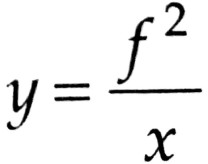is less than fthat is image always lies behind the mirror diminished in size and located between the focus and then pole.

Q.19. What is colour blindness ? How can it be cured ?

Ans ⇒ Colour Blindness : A person who cannot distinguish between various colours but can see all otherwise, is said to be colour blind. It is due to lack of some cones in the retina of the eyes. Hence cones are mainly responsible for colour blindness.
The cones which are very sensitive towards colours are made active by medical add and hence the colour blindness can be curve.

Q.20. How can spherical aberration be mini mized ?

Ans ⇒ When the rays not meeting after refraction at a point on the axis is called spherical aberration. Due to spherical aberration. The shape of the image is curved and distorted.
In order to remove (or, minimised) this error (spherical aberration) a stop is used. Due to the stop only the paraxial rays are allowed to be refrated. Due to refraction of paraxial rays the error like spherical aserration is minimized.

Q.21. How is chromatic aberration reduced ?

Ans ⇒ By achromatic combinations of lenses, chromatic aberration can be reduced.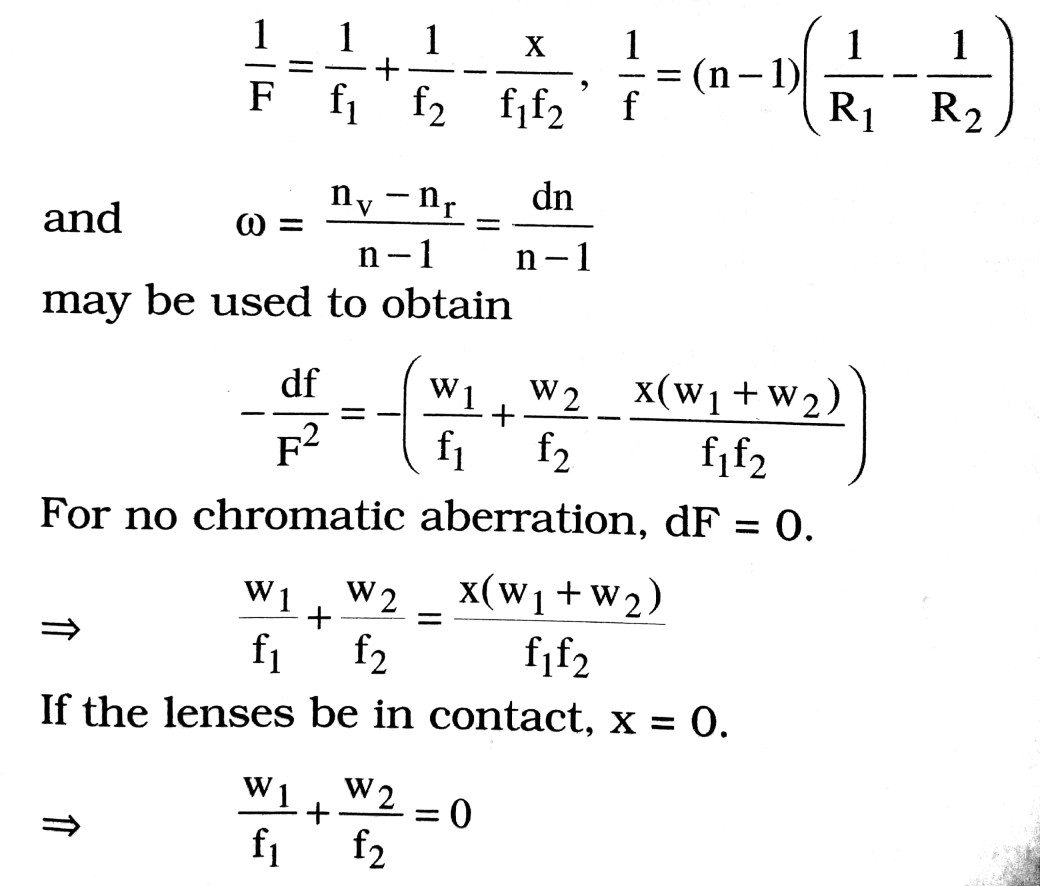One of the lenses will be converging and other diverging.

Q.22. What do you mean by power of a lens ?

Ans ⇒ The power of deviating a ray of light by a lens is inversely proportional to the focal length of the lens. It is this power which is known as the power of the lens. It is given by P = 1/f. Its S.I. unit is diopter.
The power of a lens is a characteristic of lens which does not depend upon u (distance of object) and distance of image (v).
The power of a lens is the sum of the powers of its refracting surfaces.

Q.23. What is Malus law of polarization ?

Ans ⇒ The intensity of light falls by a factor of cos20 due to transmission through a polaroid. If I. be the incident intensity and I be that beyond polaroid.
I = I0 cos2θ
where θ is the angle of plane of polarization with the plane of easy transmission in polaroid.

Q.24. What is optical fibre ? Describe its some important applications.

Ans ⇒ It is a tube of glass or quartz working on the principle of total internal reflection with the help of this device light signals are sent from one place to the other.
Applications :
(i) In field of computer or communication lines.
(ü) In two dimensional or three dimensional photo communication.
(iii) In endoscope (in medical field).
(iv) To measure rate of blood flow in heart.
(v) In refractometer.
To send a signal from one place to the other, it is first converted into light signal and then transmitted through optical fibre. Et

Q.25. Give difference between primary and secondary rainbow.

Ans ⇒ After rain water drops are suspended in air or during drizzling coloured arc of concentric circles are visible in sky. These are called rainbow. In primary rainbow violet colour of spectrum is at inner side and red colour is on outerside. The outer rainbow is secondary. It is faint in comparison to primary rainbow. Its outer edge is violet and inner edge is red. Both rainbow contain all colours.

Q.26. What is the effect of nature of medium on velocity of light ?

Ans ⇒ Nature of any medium is expressed by refractive index of medium. If refractive index of medium is μ, then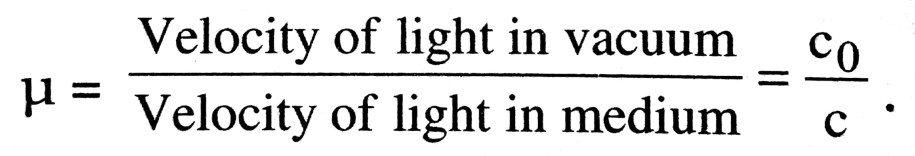So, velocity of light is inversely proportional to absolute refractive index of medium. The refractive index of dense medium is greater than the refractive index of rare medium. Velocity of light in rare medium is greater than in dense medium.

Q.27. What do you mean by retinal fatigue ?

Ans ⇒ When white object is seen after looking a bright object for long time it does not appear in its original colour. It appears with black strips. It is due to retinal fatigue. On looking bright object eyes are sensitised which is unaffected by low intensity. If a white object is seen after viewing red spot for a long time. Then it appears greenish indigo because it is complementry colour of red.

Q.28. When a lens is dipped in water its power changes. Why ?

Ans ⇒ If a lens is dipped in such a liquid whose refractive index is smaller than refractive index of material of prism then its power decreases and focal length increases but nature of lens does not change means convex lens remains convex.

Q.29. What do you mean by minimum deviation of a prism ?

Ans ⇒ The angle of deviation varies with angle of incidence. The angle of deviation has the minimum value for only one value of the angle of incidence.
The minimum value of angle of deviation when a ray of light passes through a prism is called the angle of minimum deviation. It is given by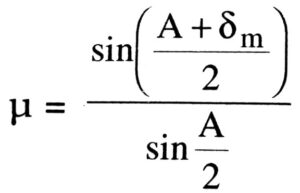where u refractive index of the materials of prism, A is angle of prism and is the angle of minimum deviation. This equation gives the relation between the refractive index of the material of the prism & angle of minimum deviation.

Q.30. What do you mean by scattering of light ?

Ans ⇒ When light beam crosses through a medium, its intensity becomes low because particles of inedium partly absorbed the light energy. Some part of energy is radiated by particle which is called scattered light, This re-radiation by particles of medium is called scattering.
By experiment, Tyndall found that light propagated by scattering medium the blue light is seen first. In course of increasing scattering particles the colour of scattered light changes. Besides it he also found that if propagate light is unpolarised then scattered light was equally polarised. This is called Tyndall scattering.

Q.31. What are advantage of pair of eye instead of single eye ?

Ans ⇒ By pair of eye there is correct estimation of distance. By one eye it is difficult to fill ink in pen, string in hole of needle. Besides these things pair of eye helps us in observing three dimensional objects. By right eye right portion of the object is seen clearly and by left part is seen. Brain adjust two parts as a whole. This combined effect help us in estimation of depth and solidness of object.

Q. 32. What is Brewster’s law ?

Ans ⇒ When unpolarized light falls on glass or water or on some other material, reflected light generally plane polarised. But at a certain angle there is complete polarization. This angle is called angle of polarization.
According to this law, tangent of angle of polarization is equal to refractive index of medium.
Refractive index of medium, μ = tan ip where ip is angle of polarization.

Q.33. A fluorescent tube of 40 watt provides more light than that of filament bulb of 40 watt. Why ?

Ans ⇒ Filament bulb is a thermal source of light from which ultraviolet & infrared radiation are emitted besides light. While inside the tube ultraviolet radiation are emitted with light. Ultraviolet radiations are modified into visible light by photo luminescent. So, efficiency of tube is more than filament bulb of same wattage.

Q.34. What are necessary conditions for obtaining pure spectrum ?

Ans ⇒ For obtaining pure spectrum following conditions should be satisfied –
(i) Slit should be narrow.
(ii) Rays coming out from convex lens should have parallel incidence.
(iii) Prism should be in condition of minimum deviation.
(iv) Emerging light rays should be focussed by achromatic lens.

Q.35. In a special case diffraction of light can be considered as interference. Explain.

Ans ⇒ Interference is the result of superposition of light waves coming from two or more sources. To study the diffraction incident wavefront on obstacle is divided into many elements and considering the superposition of light waves froin each element, we easily discuss the diffraction by obstacle. So, it is called special case of interference.

Q.36. Why does red rose appears black in presence of blue light ?

Ans ⇒ The colour of a body depends upon the kind of light reaches the eyes of observer after reflection from the surface of body. If no light is reflected by the body then body will appear black. When red rose is kept in present of blue light, blue light is absorbed by rose. So, no light is reflected. Hence rose appears black.
It also holds good for any two colours. White body reflects light of all colours in same manner. Hence they appear red in red light, green in green light etc. Bodies whether opaque or transparent have no colour of their own.

Q.37. What are necessary condition for interference ?

Ans ⇒ To obtain sustained interference pattern following conditions should be satisfied :
(i) Two light sources should be coherent i.e., two waves emitted by two sources should have constant phase difference.

(ii) The frequency or wavelength of wave obtained from two sources should be equal and light should be monochromatic.

(iii) The amplitude of two waves should be equal or nearly equal.

(iv) Both wave should travel in same direction.

(v) If light is polarised then conditions of polarised wave should be same.

Q.38. Why a soap bubble of colourless solution appears coloured in presence of white light ?

Ans ⇒ When white light is made to incident of a thin film or layer having thickness of the order of wavelength, its some path is reflected from upper surface of film & some part is reflected from inner surface of film. Due to difference in thickness of two layers a path difference is produced. When light reaches to eyes they interfere. If path difference between wave reaching on eyes for a colour is equal to one of λ/2, 3λ/2, 5λ/2.Due to destructive inteference the colour disappears in remaining light complementry colour of this colour are visible so layers is seen coloured.

Q.39. When colour glass is powdered it appears white, why ?

Ans ⇒ In case of powder coloured glass is in form of fine particles. These particles have different layers by which light is reflected continously as a result light do not go in deep layer. So very few part of light is absorbed. But in form of powder there is very few absorption of light so powder appears white or colour becomes light.

Q.40. What do you mean by persistence of vision ?

Ans ⇒ The effect of an object seen by us persist on brain upto 1/10 sec, This is called persistance of vision. When an illuminated object is whirled luminous ring appears. The time interval between two seen is less than 1/10 sec.

Q.41. All the time of sunset, the sun appears some what higher than its actual position. Why ?

Ans ⇒ Because the density of air near the earth is large and gradually decreases on going far. Due lo decrease in density refractive index of air decreases. So, the sunlight passes from rare to dense medium of spherical layers. So, they are included towards normal so the sun appears higher than actual due to refraction of light in atmosphere.

Q.42. Give difference between reflecting and refracting telescopes.

Ans ⇒ In refracting telescope only lens are used. They are generally of three types :
(a) Terrestiral (b) Astronomical & (c) Galilan.
In Galilion telescope eye piece is a concave lens. Image formed by it is also erect like terrestrial.
In reflecting telescope, objective is a large concave mirror & image by mirror is seen by eye piece. Generally all large telescope in the world one reflecting telescopes.

Q.43. What do you mean by distortion in a lens ?

Ans ⇒ Distortion in lens is that type of defect due to which ratio of magnification in different section is different. Due to this defect there is unequal magnification. It is of two types :
(i) Pin cushion distortion and (ii) Barrel distortion.
When magnification increases on increasing axial distance, distortion produced is called pin cushion type. On the other hand, magnification decreases on increasing distance, it is called Barrel type distortion.

Q.44. Why is aperture of objective kept small in compound microscope ?

Ans ⇒ The microscope is used in sighting small objects situated nearby. If aperture is large then light ray coming from object expands. So intensity becomes small. If aperture is small then light coming from object expands in small aperture, as a result object is seen more bright so aperture is kept small.

Q.45. Why does sky appear blue ?

Ans ⇒ When light energy falls on material particles, they get excited by taking energy. These excited particles again come in ground state after radiating. This process is called scattering of light. Scattering of ligh takes place in all directions. The amount of scattered light is inversely proportional to fourth power of wavelength. The wavelength of blue colour is nearly 5/9 times red component. So, blue colour is scattered 10 times more than red colour. Due to this reason sky appears blue.

Q.46. Why does the sun appear red at time of sunrise and sunset ?

Ans ⇒ At the time of sunrise and sunset sun rays have to travel larger distance through atmosphere. So, light reaches us after crossing scattering particles. During this period blue components are scattered in large amount. Due to larger wavelength light of red colour, it scattered in very less amount. Since light of red colour (red component) reaches us in large amount so sky and horizon appears red.

Q.47. Diamond appears very bright. Why?

Ans ⇒ The critical angle of diamond is 24.4°, while critical angle of simple glass is 41.8°. So, total internal reflection inside diamond is greater than simple glass, it appears bright. In deserts mirage appears and cold polar region looming appears which are result of total internal reflection.

Q.48. Explain the cause of dispersion of light.

Ans ⇒ Dispersion of light mainly depends upon nature of material or refractive index of prism which is different for lights of different colour. For red light its value is the lowest and for violet light it is greatest. When white light ray passes through a prism, from formula δ = (μ-1)A the deviation of red ray is smallest and deviation of violet ray is greatest.

Q.49. What do you mean by dispersive power ?

Ans ⇒ For any two colour the ratio of angle of dispersion and deviation for mean ray is called dispersive power of material of lens. It is generally denoted by ω.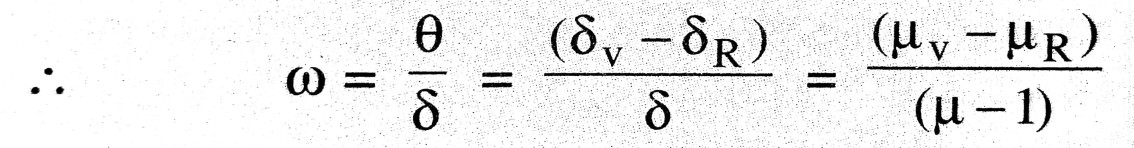The dispersive power of material of prism does not depend upon geometry of prism but nature of material of prism.

Q.50. At night, it is difficult to see objects outside from the glass window of a lighted room. If light is off then we can see easily. Explain.

Ans ⇒ The intensity of reflected light inside the room is large while light coming from outside is of negligible amount. But when source is removed then light coming from outside is appreciable and hence we see easily objects of outside.

Q.51. What do you mean by magnifying power or angular magnification ?

Ans ⇒ The ratio of angle formed on eyes by final image (R) and angle formed by object (a) kept at the same place is called magnifying power or angular magnification i.e., magnifying power, M = β/∝. It has a unit X and no dimensions. Magnifying power is also called angular magnification.

Q.62. Give the difference between emission spectrum & absorption spectrum ?

Ans ⇒ When an object is heated so much that it emits light, it is called incandescent object. Spectrum obtained from such light is called emission spectrum.

Example: Sun, carbon arc, electric bulb are source of emission spectrum.
When light rays coming from source producing continuous spectrum are passed through transparent solids, liquid or gases, objects absorb some part of light. Solar spectrum is an example of absorption spectrum.

## Class 12th physics Subjective question in English

 S.N Physics Short Type Question English Medium 1. ELECTRIC CHARGES AND FIELDS 2. LECTROSTATIC POTENTIAL AND CAPACITANCE 3. CURRENT ELECTRICITY 4. MOVING CHARGES AND MAGNETISM 5. MAGNETISM AND MATTER 6. ELECTROMAGNETIC INDUCTION 7. ALTERNATING CURRENT 8. ELECTROMAGNETIC WAVES 9. RAY OPTICS AND OPTICAL INSTRUMENTS 10. WAVE OPTICS 11. DUAL NATURE OF MATTER AND RADIATION 12. ATOMS 13. NUCLEI 14. SEMI CONDUCTOR ELECTRONICS 15. COMMUNICATION SYSTEMS